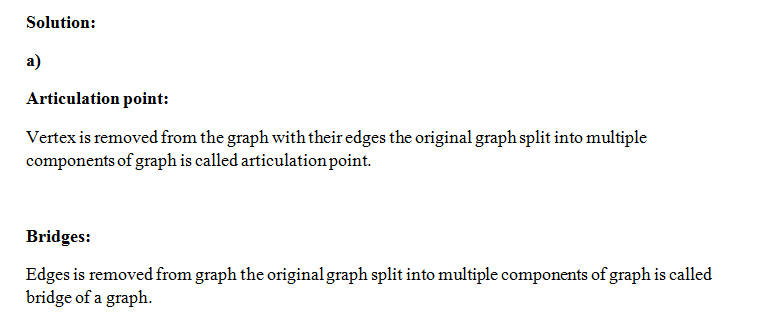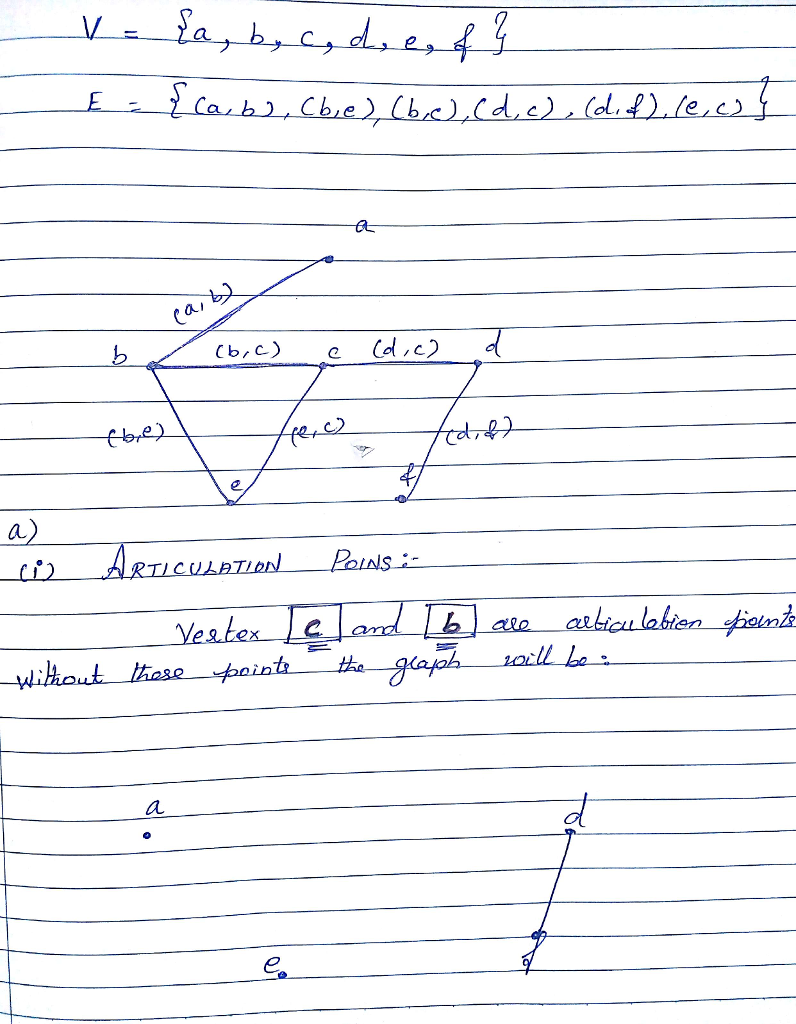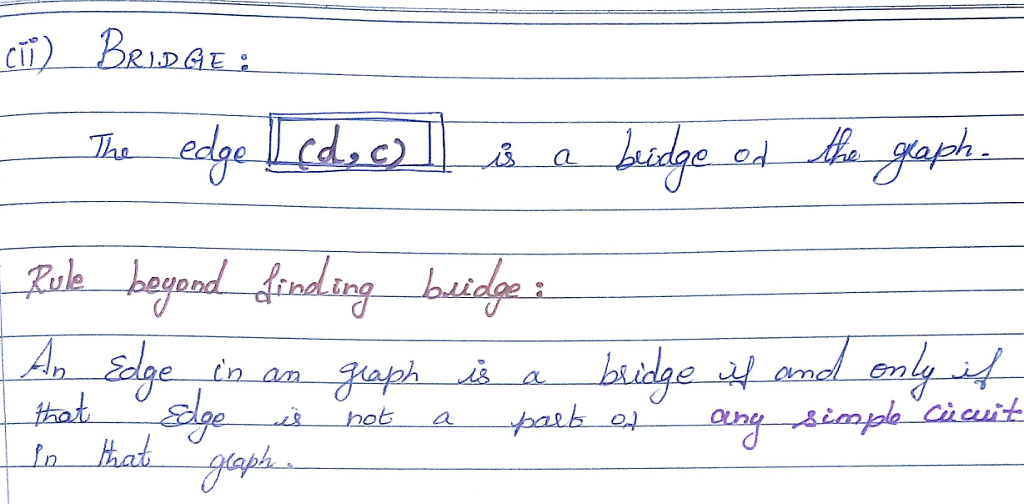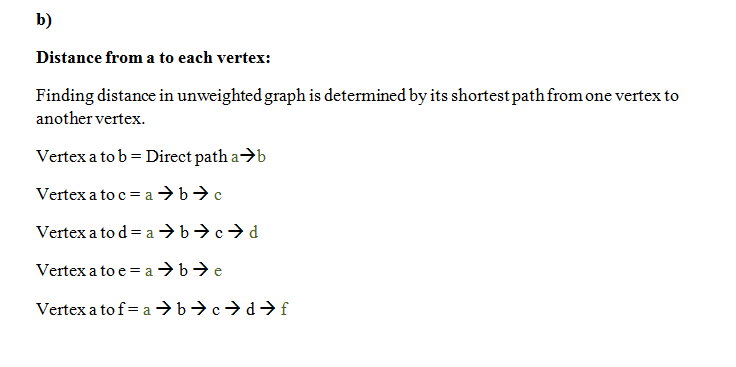# Question & Answer: Undirected Graphs Let G = (V, E) be an undirected graph, where V = {a, b, c, d, e, f} an…..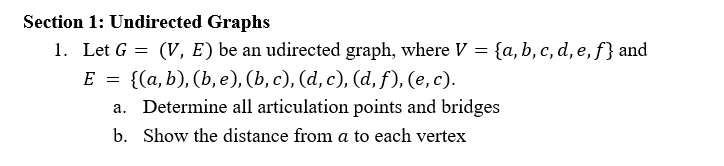Undirected Graphs Let G = (V, E) be an undirected graph, where V = {a, b, c, d, e, f} and E = {(a, b), (b, e), (b, c), (d, c), (d, f), (e, c). a. Determine all articulation points and bridges b. Show the distance from a to each vertex